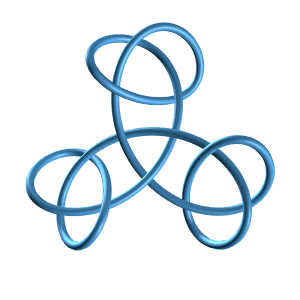#### Tube: Crossing Knot Pair

Template: Graphics/3d-tube-shape

Discription: This template creates a crossing knot pair tube that extrudes along a 3D curve based on a set of parametric equations x(t), y(t), and z(t).

Price: \$10.00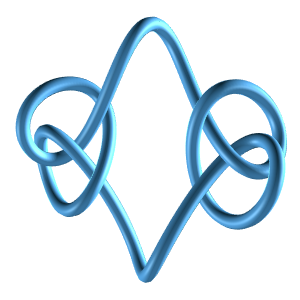#### Tube: Crossing Knot Pair 2

Template: Graphics/3d-tube-shape

Discription: This template creates another crossing knot pair tube that extrudes along a 3D curve based on a set of parametric equations x(t), y(t), and z(t).

Price: \$10.00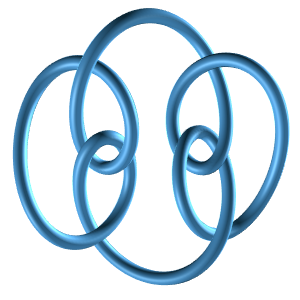#### Tube: Granny Knot Pair

Template: Graphics/3d-tube-shape

Discription: This template creates a Granny knot pair tube that extrudes along a 3D curve based on a set of parametric equations x(t), y(t), and z(t).

Price: \$10.00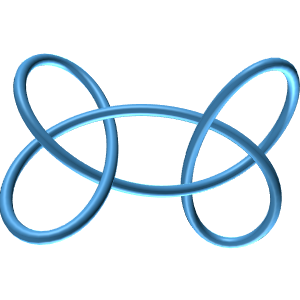#### Tube: Lissajous Knot

Template: Graphics/3d-tube-shape

Discription: This template creates various Lissajous knot tubes that extrude along a 3D curve based on a set of parametric equations x(t), y(t), and z(t) by specifying different sets of parameters.

Price: \$15.00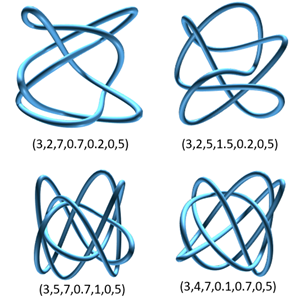#### Tube: Polynomial Knot (5,1)

Template: Graphics/3d-tube-shape

Discription: This template creates a polynomial (5,1) knot tube that extrudes along a 3D curve based on a set of parametric equations x(t), y(t), and z(t).

Price: \$10.00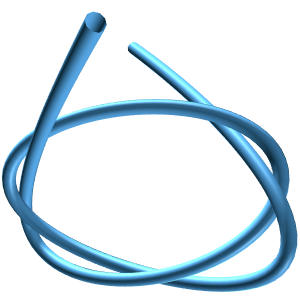#### Tube: Polynomial Knot (6,2)

Template: Graphics/3d-tube-shape

Discription: This template creates a polynomial (6,2) knot tube that extrudes along a 3D curve based on a set of parametric equations x(t), y(t), and z(t).

Price: \$10.00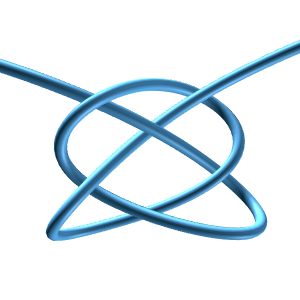#### Tube: Polynomial Knot (7, 4)

Template: Graphics/3d-tube-shape

Discription: This template creates a polynomial (7,4) knot tube that extrudes along a 3D curve based on a set of parametric equations x(t), y(t), and z(t).

Price: \$10.00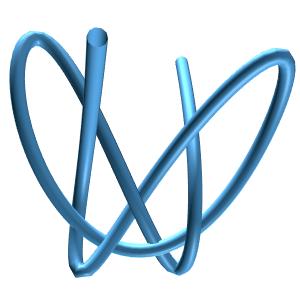Template: Graphics/3d-tube-shape

Discription: This template creates a quadruple knot pair tube that extrudes along a 3D curve based on a set of parametric equations x(t), y(t), and z(t).

Price: \$10.00#### Tube: Square Knot Pair

Template: Graphics/3d-tube-shape

Discription: This template creates a square knot pair tube that extrudes along a 3D curve based on a set of parametric equations x(t), y(t), and z(t).

Price: \$10.00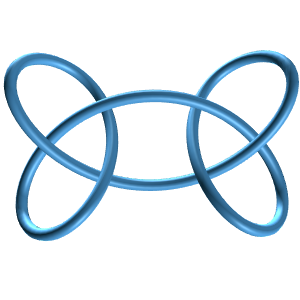#### Tube: Torus Knot

Template: Graphics/3d-tube-shape

Discription: This template creates various torus knot tubes that extrude along a 3D curve based on a set of parametric equations x(t), y(t), and z(t) by specifying different sets of parameters.

Price: \$15.00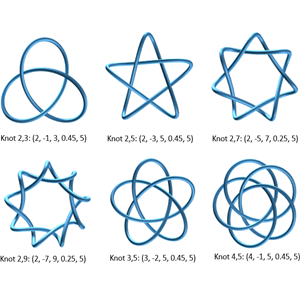#### Tube: Trefoil Knot

Template: Graphics/3d-tube-shape

Discription: This template creates various Trefoil knots that extrude along a 3D curve based on a set of parametric equations x(t), y(t), and z(t) by specifying different sets of parameters.

Price: \$15.00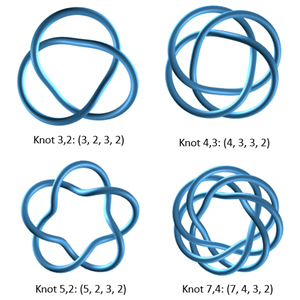#### Tube: Triple Knot Pair

Template: Graphics/3d-tube-shape

Discription: This template creates a triple knot pair tube that extrudes along a 3D curve based on a set of parametric equations x(t), y(t), and z(t).